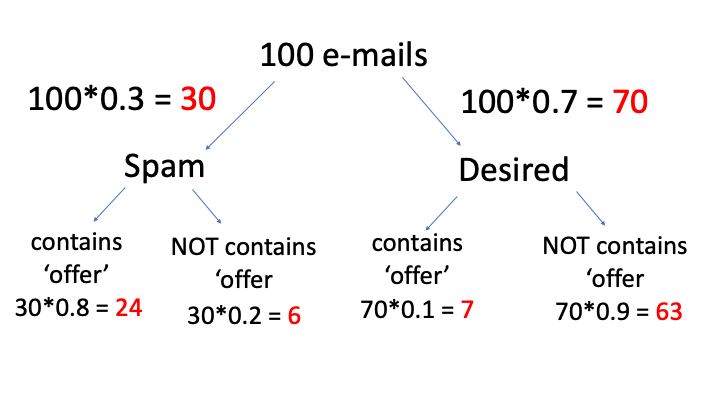# Bayes Theorem

## Definition

Bayes theorem is also known as the formula for the probability of causes.

Theorem states that the conditional probability of an event, based on the occurrence of another event, is equal to the likelihood of the second event given the first event multiplied by the probability of the first event.

## Conditional Probability

Two events A and B from the same sample space S. Calculate the probability of event A knowing that event B has occurred. B is the “conditioning event”. $$P(A|B)$$

Conditional Probability is $$P(A \mid B)=\frac{P(A \cap B)}{P(B)}, \quad P(B)>0$$

## Multiplication Rule

This leads to the multiplication rule $$P(A \cap B) = P(B) P(A \mid B) = P(A) P(B \mid A)$$

## Bayes Theorem

Bayes Theorem $$P(A \mid B) = \frac{P(B \mid A)P(A)} {P(B)}$$

### Example

Bayes Theorem can detect spam e-mails.

• Assume that the word offer occurs in 80% of the spam messages.

• Also assume offer occurs in 10% of my desired e-mails.

Question

If 30% of the received e-mails are considered as a scam, and I will receive a new message which contains ‘offer’, what is the probability that it is spam?

• I have 30 spam e-mails

• 70 desired e-mails.

The percentage of the word ‘offer’ that occurs in spam e-mails is 80%. It means 80% of 30 e-mail and it makes 24. Now, I know that 30 e-mails of 100 are spam and 24 of them contain ‘offer’ where 6 of them not contains ‘offer’.

The percentage of the word ‘offer’ that occurs in the desired e-mails is 10%. It means 7 of them (10% of 70 desired e-mails) contain the word ‘offer’ and 63 of them not.The question was what is the probability of spam where the mail contains the word ‘offer’:

1. We need to find the total number of mails which contains ‘offer’ ; 24 +7 = 31 mail contain the word ‘offer’

2. Find the probability of spam if the mail contains ‘offer’ ;

In 31 mails 24 contains ‘offer’ means 77.4% = 0.774 (probability)

NOTE: In this example, I choose the percentages which give integers after calculation. As a general approach, you can think that we have 100 units at the beginning so if the results are not an integer, it will not create a problem. Such that, we cannot say 15.3 e-mails but we can say 15.3 units.

Solution with Bayes’ Equation:

A = Spam

B = Contains the word ‘offer’

$P(\text { spam } \mid \text { contains offer })=\frac{P(\text { contains offer } \mid \text { spam }) * P(\text { spam })}{P(\text { contains offer })}$

P( contains offer|spam) = 0.8 (given in the question)

P(spam) = 0.3 (given in the question)

Now we will find the probability of e-mail with the word ‘offer’. We can compute that by adding ‘offer’ in spam and desired e-mails. Such that;

P(contains offer) = 0.30.8 + 0.70.1 = 0.31

$P(\text { spam } \mid \text { contains offer })=\frac{0.8 * 0.3}{0.31}=0.774$

As it is seen in both ways the results are the same. In the first part, I solved the same question with a simple chart and for the second part, I solved the same question with Bayes’ theorem.

## Law of Total Probability

$$B=(B \cap A) \cup\left(B \cap A^{c}\right)$$

$$P(B)=P(B \cap A)+P\left(B \cap A^{c}\right)=P(B \mid A) P(A)+P\left(B \mid A^{c}\right) P\left(A^{c}\right)$$

## Independence and Mutually Exclusive Events

Two events are independent if knowing the outcome of one event does not change the probability of the other.

• Flip a two-sided coin repeatedly. Knowing the outcome of one flip does not change the probability of the next.

Two events, A and B, are independent if $$P(A|B) = P(A)$$, or equivalently $$P(B|A) = P(B)$$.

Recall: $$P(A \mid B)=\frac{P(A \cap B)}{P(B)}$$

then, if A and B are independent, we get the multiplication rule for independent events:

$$P(A \cap B)=P(A) P(B)$$

### Example

Suppose your company has developed a new test for a disease. Let event A be the event that a randomly selected individual has the disease and, from other data, you know that 1 in 1000 people has the disease. Thus, P(A) = .001. Let B be the event that a positive test result is received for the randomly selected individual. Your company collects data on their new test and finds the following:

• $$P(B|A)$$ = .99

• $$P(B^c |A)$$ = .01

• $$P(B|A^c )$$ = .02

Calculate the probability that the person has the disease, given a positive test result. That is,

find $$P(A|B)$$.

\begin{split} \begin{aligned} P(A \mid B) &=\frac{P(A \cap B)}{P(B)} \\ &=\frac{P(B \mid A) P(A)}{P(B)} \leftarrow \text { Bayes theorem } \\ &=\frac{ P(B\mid A) P(A)}{P(B \mid A) P(A)+P\left(B \mid A^{c}\right) P\left(A^{c}\right)} \leftarrow \text { Law of Total Probability } \\ &=\frac{(.99)(.001)}{(.99)(.001)+(.02)(.999)} \\ &=.0472 \end{aligned} \\ P(A) =.001 \leftarrow \text { prior prob of } A \\ P(A \mid B) =.0472 \leftarrow \text { posterior prob of } A \end{split}